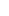You use margin to create leverage.

Leverage is the increased “trading power” that is available when using a margin account.

We express leverage as a ratio.

Leverage is the ratio between the amount of money you really have and the amount of money you can trade.

They usually expressed it in an “X:1” format.

For example, if you wanted to trade 1 standard lot of USD/JPY without margin, you would need \$100,000 in your account.

But with a Margin Requirement of just 1%, you would only have to deposit \$1,000 in your account.

The leverage provided for this trade would be 100:1.

## Example

Here are examples of Leverage Ratios depending on the Margin Requirement:

 Currency Pair Margin Requirement Leverage Ratio EUR/USD 2% 50:1 GBP/USD 5% 20:1 USD/JPY 4% 25:1 EUR/AUD 3% 33:1

Here’s how to calculate Leverage:

`Leverage = 1 / Margin Requirement`

For example, if the Margin Requirement is 2%, here’s how to calculate leverage:

`50 = 1 / .02`

The leverage is 50, which is expressed as a ratio, 50:1

Here’s how to calculate the Margin Requirement based on the Leverage Ratio:

`Margin Requirement = 1 / Leverage Ratio`

For example, if the Leverage Ratio is 100:1, here’s how to calculate the Margin Requirement.

`0.01 = 1 / 100`

The Margin Requirement is 0.01 or 1%.

As you can see, leverage has an inverse relationship to margin.

“Leverage” and “margin” refer to the same concept, just from a slightly different angle.

When a trader opens a position, they are required to put up a fraction of that position’s value “in good faith”. In this case, the trader is said to be “leveraged”.

The “fraction” part which is expressed in percentage terms is known as the “Margin Requirement”. For example, 2%.

We know the actual amount that is required to be put up as the “Required Margin”.

For example, 2% of a \$100,000 position size would be \$2,000.

The \$2,000 is the Required Margin to open this specific position.

Since you are able to trade a \$100,000 position size with just \$2,000, your leverage ratio is 50:1.

```Leverage = 1 /Margin Requirement

50 = 1 / 0.02```